# Inequalities Involving Absolute Values

Inequalities Involving Absolute Values

The absolute value of any number gives us the magnitude of that number. That is, if a number x  is positive, its absolute value will be x itself, while if x is negative, then its absolute value will be –x, which is positive. The absolute value of 0 is 0. The absolute value is denoted by two vertical bars put around the relevant number. Here are some examples:

$\begin{array}{l}\left|3 \right| = 3 & & \left| { - 3} \right| = 3 & \\\left|{\sqrt 2 } \right| = \sqrt 2 & & \left| { - \sqrt 2 } \right| = \sqrt2 \\\left| {7 - 11} \right| = 4 & & \left| {1 - \pi } \right| = \pi -1\\\left| {\pi - 1} \right| = \pi - 1 & & \left| 0 \right| =0\end{array}$

The absolute value of a number measures the distance of that number from the point 0. And since distances are always non-negative, the absolute value of any number will always be non-negative. Here are two examples where we interpret the absolute value of a number as its distance from the point 0: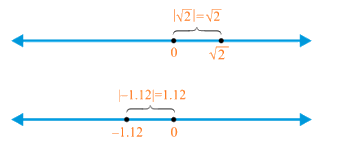Consider the following equation involving an absolute value:

$\left| x \right| =3$

How many numbers x will satisfy this equation? How many numbers are there such that their distance from the point 0 is 3? There are exactly two such points, 3 and –3:Thus, the solution to this equation is

$x = \pm 3$

The plus minus sign denotes that there are two solutions, one corresponding to the plus sign and one to the minus sign. Now, consider the following equation:

$\left| {x - 1}\right| = 3$

What is the solution to this equation? We have

$\begin{array}{l}x -1 = \pm 3\\ \Rightarrow \;\;\;x = 1 \pm 3 = 4,\; - 2\end{array}$

Thus, there are two solutions: 4 and –2. The way this equation should be interpreted is important. Just as the absolute value of x denotes the distance of x from the point 0,the absolute value of $$x - 1,$$ which is represented as $$\left| {x - 1} \right|$$, should be interpreted as the distance of the point x from the point 1. Thus, the equation

$\left|{x - 1} \right| = 3$

says that x is a number such that the distance of x from the point 1 is 3. How many such numbers are there? Exactly two: 4 and –2. This is clear from the diagram below: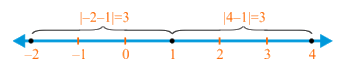We will now consider simple linear inequations involving absolute values. Consider the following inequation:

$\left| x \right|> 3$

What does this inequation say? It says that x is a number such that the distance of x from the point 0 is greater than 3. Visualize carefully: where can x lie on the number line?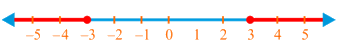x can lie anywhere on the red part(s) of the number line in the diagram above. This is because if x  is anywhere on this part of the number line (whether on the positive side or the negative side), its distance from 0 will always be greater than 3. Convince yourself about this point. Thus, the solution to this particular inequation is

$x >3\;\;{\rm{or}}\;x < - 3$

Whenever you encounter absolute values, it is important to think in terms of distances. This way of thinking will really help simplify things when you go on to study complex numbers. Let’s take another example. Consider the inequation

$\left| {x + 1}\right| \le 2$

We can rewrite this as

$\left| {x - \left( {- 1} \right)} \right| \le 2$

This equation now says that the distance of the point x from the point –1 is less than or equal to 2. Where can x lie on the number line such that it satisfies this constraint? Consider the following diagram: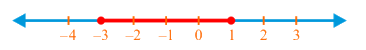Note carefully that if lies anywhere on the red part of the number line above, then its distance from –1 must be less than (or equal to) 2, but never greater than 2.This means that the solution to this inequation is

$- 3\le x \le 1$

Example 1: Determine the solution to the following inequation, and plot the solution set on a numberline:

$\left| {x - \sqrt 2} \right| > 1$

Solution: This inequation says that the distance of x from the point $$\sqrt2$$ is greater than 1: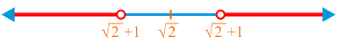In the figure above, if lies anywhere on the red part of the number line, its distance from $$\sqrt 2$$ will be greater than 1. Thus, the solution to this inequation is

$x > \sqrt 2 +1\;\;{\rm{or}}\;\;x < \sqrt 2 - 1$

Example 2: Determine the solution to the following inequation, and plot the solution set on a number line:

$\left| {2x + \pi }\right| \le 4$

Solution: We can rewrite this inequation as follows:

$\begin{array}{l}\left|{2\left( {x + \frac{\pi }{2}} \right)} \right| \le 4\\ \Rightarrow \;\;\;\left|{x + \frac{\pi }{2}} \right| \le 2\\ \Rightarrow \;\;\;\left| {x - \left( { -\frac{\pi }{2}} \right)} \right| \le 2\end{array}$

This inequation now says that the distance of the point x from the point $$- \frac{\pi }{2}$$ is less than or equal to 2. The solution set can be easily plotted, as follows:We can now write the solution to this inequation as

$- \frac{\pi }{2} -2 \le x \le - \frac{\pi }{2} + 2$

Linear Equations
Linear Equations
grade 9 | Questions Set 2
Linear Equations
Linear Equations
grade 9 | Questions Set 1
More Important Topics
Numbers
Algebra
Geometry
Measurement
Money
Data
Trigonometry
Calculus
More Important Topics
Numbers
Algebra
Geometry
Measurement
Money
Data
Trigonometry
Calculus